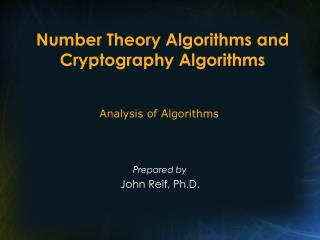DownloadDownload PresentationNumber Theory Algorithms and Cryptography Algorithms

# Number Theory Algorithms and Cryptography Algorithms

Download Presentation## Number Theory Algorithms and Cryptography Algorithms

- - - - - - - - - - - - - - - - - - - - - - - - - - - E N D - - - - - - - - - - - - - - - - - - - - - - - - - - -
##### Presentation Transcript

1. Number Theory Algorithms and Cryptography Algorithms Analysis of Algorithms Prepared by John Reif, Ph.D.

2. Number Theory Algorithms • GCD • Multiplicative Inverse • Fermat & Euler’s Theorems • Public Key Cryptographic Systems • Primality Testing

3. Number Theory Algorithms (cont’d) • Main Reading Selections: • CLR, Chapter 33

4. Euclid’s Algorithm • Greatest Common Divisor • Euclid’s Algorithm

5. Euclid’s Algorithm (cont’d) • Inductive proof of correctness:

6. Euclid’s Algorithm (cont’d) • Time Analysis of Euclid’s Algorithm for n bit numbers u,v

7. Euclid’s Algorithm (cont’d) • Fibonacci worst case:

8. Euclid’s Algorithm (cont’d) • Improved Algorithm

9. Extended GCD Algorithm

10. Extended GCD Algorithm (cont’d) • Theorem • Proof

11. Extended GCD Algorithm (cont’d) • Corollary If gcd(x,y) = 1 then x' is the modular inverse of x modulo y • Proof

12. Modular Laws • Gives Algorithm for • Modular Laws

13. Modular Laws (cont’d)

14. Modular Laws (cont’d)

15. Fermat’s Little Theorem • If n prime then an = a mod n • Proof by Euler

16. Fermat’s Little Theorem (cont’d)

17. Euler’s Theorem • Φ(n) = number of integers in {1,…, n-1} relatively prime to n • Euler’s Theorem • Proof

18. Euler’s Theorem (cont’d) • Lemma • Proof

19. Euler’s Theorem (cont’d) • By Law A and Lemma • By Law B

20. Taking Powers mod n by “Repeated Squaring” • Problem: Compute ae mod b

21. Rivest, Sharmir, Adelman (RSA) Encryption Algorithm • M = integer message e = “encryption integer” for user A • Cryptogram

22. Rivest, Sharmir, Adelman (RSA) Encryption Algorithm (cont’d) • Method

23. Rivest, Sharmir, Adelman (RSA) Encryption Algorithm (cont’d) • Theorem

24. Rivest, Sharmir, Adelman (RSA) Encryption Algorithm (cont’d) • Proof

25. Rivest, Sharmir, Adelman (RSA) Encryption Algorithm (cont’d) • By Euler’s Theorem

26. Security of RSA Cryptosystem • Theorem If can compute d in polynomial time, then can factor n in polynomial time • Proof e· d-1 is a multiple of φ(n) But Miller has shown can factor n from any multiple of φ(n)

27. Security of RSA Cryptosystem (cont’d)

28. Rabin’s Public Key Crypto System • Use private large primes p, q public key n=q p message M cryptogram M2 mod n • Theorem If cryptosystem can be broken, then can factor key n

29. Rabin’s Public Key Crypto System (cont’d) • Proof • In either case, two independent solutions for M give factorization of n, i.e., a factor of n is gcd (n, γ -β).

30. Rabin’s Public Key Crypto System (cont’d) • Rabin’s Algorithm for factoring n, given a way to break his cryptosystem.

32. Jacobi Function

33. Jacobi Function (cont’d) • Gauss’s Quadratic Reciprocity Law • Rivest Algorithm

34. Jacobi Function (cont’d) • Theorem (Fermat)

35. Theorem: Primes are in NP • Proof

36. Primality Testing • Testing • Goal of Randomized Primality Testing

37. Primality Testing (cont’d) • Solovey & Strassen Primality Test quadratic reciprocal law

38. Definitions

39. Theorem of Solovey & Strassen • Theorem • Proof

40. Theorem of Solovey & Strassen (cont’d)

41. Theorem of Solovey & Strassen (cont’d) • Then by Chinese Remainder Theorem, • Since a is relatively prime to n,

42. Theorem of Solovey & Strassen (cont’d)

43. Theorem of Solovey & Strassen (cont’d)

44. Theorem of Solovey & Strassen (cont’d)

45. Miller • Miller’s Primality Test

46. Miller (cont’d) • Theorem (Miller) Assuming the extended RH, if n is composite, then Wn(a) holds for some a ∈ {1,2,…, c log 2 n} • Miller’s Test assumes extended RH (not proved)

47. Number Theory Algorithms and Cryptography Algorithms Analysis of Algorithms Prepared by John Reif, Ph.D.